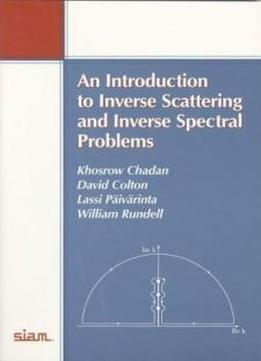# An Introduction To Inverse Scattering And Inverse Spectral Problems (monographs On Mathematical Modeling And Computation) by David Colton / 1987 / English / PDF

Here is a clearly written introduction to three central areas of inverse problems: inverse problems in electromagnetic scattering theory, inverse spectral theory, and inverse problems in quantum scattering theory. Inverse problems, one of the most attractive parts of applied mathematics, attempt to obtain information about structures by nondestructive measurements. Based on a series of lectures presented by three of the authors, all experts in the field, the book provides a quick and easy way for readers to become familiar with the area through a survey of recent developments in inverse spectral and inverse scattering problems. In the opening chapter, Paivarinta collects the mathematical tools needed in the subsequent chapters and gives references for further study. Colton's chapter focuses on electromagnetic scattering problems. As an application he considers the problem of detecting and monitoring leukemia. Rundell's chapter deals with inverse spectral problems. He describes several exact and algorithmic methods for reconstructing an unknown function from the spectral data. Chadan provides an introduction to quantum mechanical inverse scattering problems. As an application he explains the celebrated method of Gardner, Greene, Kruskal, and Miura for solving nonlinear evolution equations such as the Korteweg_de Vries equation. Each chapter provides full references for further study.

Here is a clearly written introduction to three central areas of inverse problems: inverse problems in electromagnetic scattering theory, inverse spectral theory, and inverse problems in quantum scattering theory. Inverse problems, one of the most attractive parts of applied mathematics, attempt to obtain information about structures by nondestructive measurements. Based on a series of lectures presented by three of the authors, all experts in the field, the book provides a quick and easy way for readers to become familiar with the area through a survey of recent developments in inverse spectral and inverse scattering problems. In the opening chapter, Paivarinta collects the mathematical tools needed in the subsequent chapters and gives references for further study. Colton's chapter focuses on electromagnetic scattering problems. As an application he considers the problem of detecting and monitoring leukemia. Rundell's chapter deals with inverse spectral problems. He describes several exact and algorithmic methods for reconstructing an unknown function from the spectral data. Chadan provides an introduction to quantum mechanical inverse scattering problems. As an application he explains the celebrated method of Gardner, Greene, Kruskal, and Miura for solving nonlinear evolution equations such as the Korteweg_de Vries equation. Each chapter provides full references for further study.

views: 592